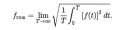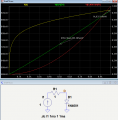Gain in class D amplifier and output power

leo0001

Joined Sep 30, 2018
79
Hello,

I m back again for understanding. I can't understand how Irms is different of Iavg. Ok there is a high peak current. If Iavg = 1 A and if the diode are conducting between t1 and T with T the period. Ipeak = Iavg*T/(T-t1) = Iavg/(1-t1/T). If t1/T is equal to 0.8. So Ipeak is 5 A. However Irms is equal to the following expression for a periodic signal :If you resolve it you will have something like this sqrt(1/T*Ipeak²*(T-t1), then sqrt[Iavg² *T/²(T-t1)²*(T-t1)] and so sqrt(Iavg²/(1-t1/T)) and the limit of this tends to Iavg. It seems to be normal to me that Iavg is equal to Irms ? Did i do a mistake ?

So diodes have no reason to heat more than they "should" do ?

Thanks,

kubeek

Joined Sep 20, 2005
5,770
I am off college for too long to be integrating anything or find your error, so lets try with concrete numbers. Say you have a square wave, where you have 1A for a quarter of the period, and a zero for the remaining three quarters. That is 0.25A average, right?

Now square root, of average, of squares is? sqrt((1^2*1+0^2*3)/4)= 0.5A.

So a wave like this has average current 0.25A, but RMS current 0.5A. And the RMS current is what heats up the diode, beacuse joule heat is I^2*R, meaning that average power dissipation is proportional to the average of squared current.

•leo0001

crutschow

Joined Mar 14, 2008
28,521
And the RMS current is what heats up the diode, beacuse joule heat is I^2*R, meaning that average power dissipation is proportional to the average of squared current.
I don't mean to muddy the discussion, but that applies only if the diode voltage drop is pure resistive, which it is not.
A diode looks more like a constant voltage drop, with a slow exponential change with current, so its dissipation is more closely proportional to the current than the square of the current.
There is a small series parasitic resistance in a diode and the dissipation due to that is, of course, proportional to the current squared.

But the dissipation in the transformer is proportional the the square of the current since the winding impedance is a pure resistance.

The difference is show in the LTspice simulation below for a typical diode:
Note that the diode dissipation (green trace) is nearly linear with the change in current, while the resistor dissipation (red trace) is clearly non-linear and proportional to the square of the current (as expected).Last edited:
•leo0001 and kubeek

kubeek

Joined Sep 20, 2005
5,770
Thank you for the clarification, somehow forgot to think about that diode vs resistor.

Ok there was an error in my computation. Sorry ... I agree with youAnd thanks for your explanation !